IF YOU CAN SEE THIS go to /sfa/Nuprl/Shared/Xindentation_hack_doc.html

### Extracting Witnesses from Proofs of Propositions

As is pointed out in Propositions as Types, the notations used in Nuprl for propositions actually denote types which are inhabited just when the corresponding propositions are true. See Propositions as Types - standard defs. Indeed, Def Prop == Type. Thus, all Nuprl Sequents are Sequents with Type Conclusions, hypotheses of sequents are declarations, and Proof Witness Extraction can be effected for all sequents.

For example, because "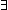x: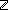x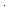x = 4" expands by definition to "x: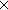xx = 4" from a proof of it one can extract an expression containing a witness (see Propositions as Types). Here's what we get from this particular proof, named sfa doc simple existential 1 incidentally:

ext{sfa_doc_simple_existential_1} *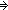<-2,*>

The first component -2 is the witness, and the second component "*" is the trivial member of any Equality Type when the equality actually holds. The equality type is empty when it doesn't hold, so one could not extract from any proof ofx:xx = 4 (i.e. x:xx = 4) a pair where the square of the first component was not 4.

Consider a more complex example. The expression "x,y:x<y & (z:z<x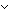y<z)" expands by definition to the type expression "x:y:(x<y)(z:(z<x)+(y<z))". Here is an extract from a proof sfa doc simple existential 2 of this proposition:

ext{sfa_doc_simple_existential_2} *<4,6,*,(-1),inl(*)>

Like equality types, a Less-than Type is defined as a primitive type that has a (trivial) member just if the relation holds between the specified integers. Observe that the last component of the tuple, inl(*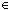(-1<4)+(6<-1) informs us that the left disjunct that is inhabited by *.

Now we move to a more interesting case. Consider Thm*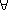x: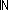y:yy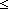x & x<(y+1)(y+1), which claims we can find a certain integer approximation of the square root of any natural number. This expression expands to "x: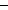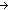y:((x<yy)Void)(x<(y+1)(y+1))", expressing the part of constructive content to the effect that there is a function for producing the witness y for each instance x of the universal. Thus, the extract of this theorem, ext{sfa_doc_nat_sqrt}, must be a function producing for each input a pair with a witness as the first component.

ext{sfa_doc_nat_sqrt}
*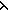x.ind(xzzj,%.%2.*%.<0,(.*),*>; zzj,%.%2.%(.*)/y,%3.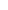x.%3/%4,%5.x.InjCase(if zzj<((y+1)(y+1))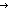inl(*) ; inr(.*) fix.InjCase%. <y,(.*),*>x.InjCase%7. <(y+1),(.*),*>))x.(.*)

Pretty ugly, but automatically extracted, and applicable: ext{sfa_doc_nat_sqrt}(5) *<2,(.*),*>.

So, 2 approximates the square root of 5. Let's evaluate this function for several values, mapping over a list, and pairing each input with the witness gotten by projecting off the result of applying ext{sfa_doc_nat_sqrt}. Def map(f;as) == Case of as; nilnil ; a.as'f(a).map(f;as')  (recursive)

map(x.<x,ext{sfa_doc_nat_sqrt}(x).1>;[0; 1; 2; 4; 5; 7; 9; 11])
*[<0,0>; <1,1>; <2,1>; <4,2>; <5,2>; <7,2>; <9,3>; <11,3>]

(Sept 2001 - sfa )